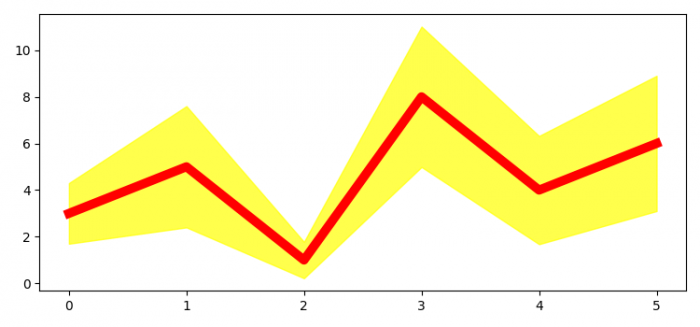# How to plot a line in Matplotlib with an interval at each data point?

To plot a line in Matplotlib with an interval at each data point, we can take the following steps −

• Set the figure size and adjust the padding between and around the subplots.
• Make an array of means and standard deviations.
• Plot means using plot() method.
• Fill the area between means+stds and means-stds, alpha=0.7 and color='yellow'.
• To display the figure, use show() method.

## Example

import numpy as np
import matplotlib.pyplot as plt

plt.rcParams["figure.figsize"] = [7.50, 3.50]
plt.rcParams["figure.autolayout"] = True

means = np.array([3, 5, 1, 8, 4, 6])
stds = np.array([1.3, 2.6, 0.78, 3.01, 2.32, 2.9])

plt.plot(means, color='red', lw=7)

plt.fill_between(range(6), means - stds, means + stds, alpha=.7, color='yellow')

plt.show()

## Output